# Bipolar Images

Two images of the same scene are shown below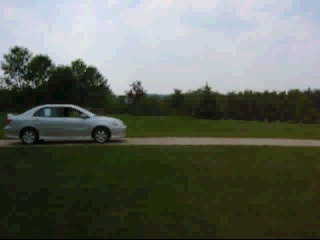Careful examination shows that the automobile moves slightly between the two images. Normal images (converted to gray-scale and normalized) vary from zero to one, where zero is black, one is white, and intermediate values are gray.

If these images are subtracted, the resulting values range from a minimum of −1 to a maximum of +1. Values less than zero will be displayed as black.

### Contrast Stretching

If `diff = g2 - g1`, where `g1` and `g2` are the grayscale images, we can rescale the difference to the range 0 – 1 by calculating

`g = (diff+1)/2`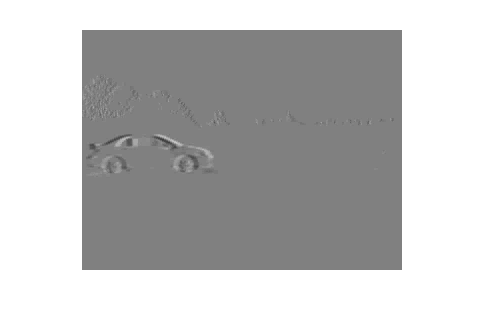Zero-difference values are medium gray. Negative differences are darker (toward black) and positive differences lighter (toward white).

The MATLAB function `bipolar_image.m` does this with `g = bipolar_image(diff,0)`.

### Color coding (black background)

Here we code negative values as shades of blue, and positive values as shades of red. Black is the zero difference reference.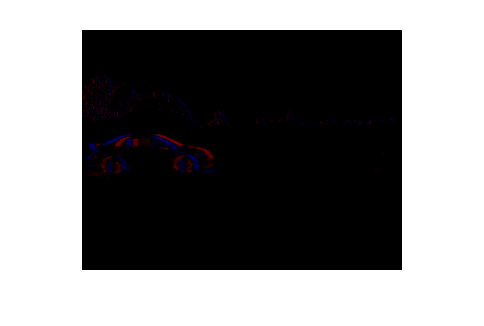The MATLAB function `bipolar_image.m` does this with `g = bipolar_image(diff,1)`.

### Color coding (white background)

We again code negative values as shades of blue, and positive values as shades of red. Now, however, white is the zero difference reference.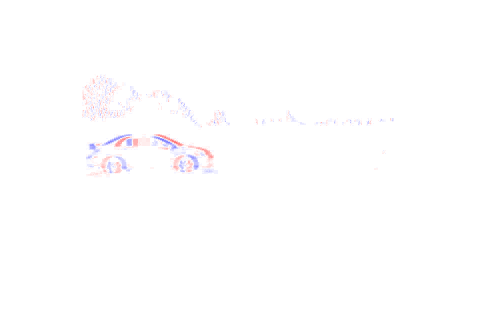The MATLAB function `bipolar_image.m` does this with `g = bipolar_image(diff,2)`.

### Color coding (image background)

We still code negative values as shades of blue, and positive values as shades of red. Values where `abs(diff)<0.3` use the grayscale values of the first image.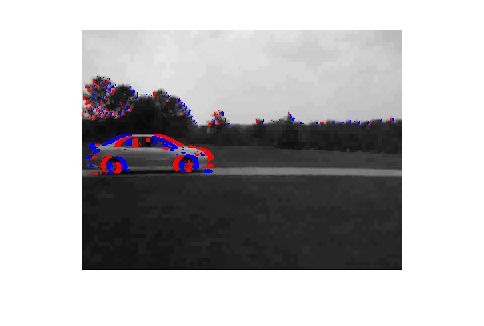The MATLAB function `imdiff.m` does this with `g = imdiff(g1,g2)`.

## Code Examples

• bp1.m, using the above images.
• bp1v.m, using target board images.